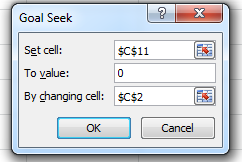# A 10 Min Tutorial On How Goal Seek WorksBusinesses are all about decision making. If you are working for a firm, whether it is producing goods or providing services, you must have come across situation where you need to do the “What-If” Analysis. One of the most helpful tools in this context is “Goal Seek”.

The name is self-explanatory as the tools helps to reach us a goal by changing certain variable. This tools simply removes the process of iteration that consumes lot of time. The tool can be accessed through Data>What If Analysis>Goal Seek or by using shortcut keys: Alt+A+W+G.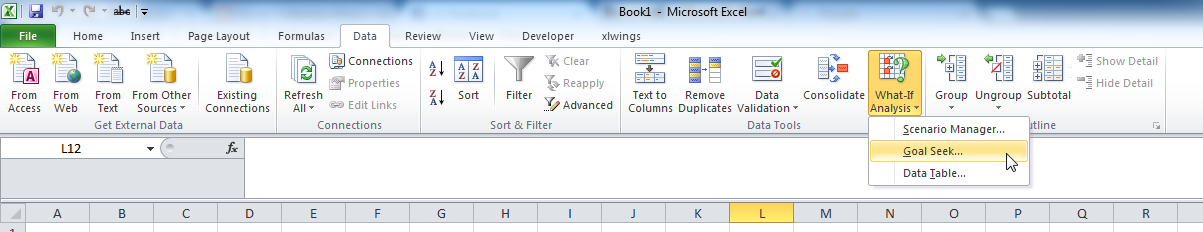The Goal-Seek comes with three options: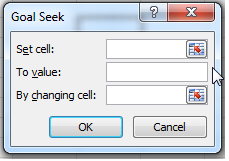Set Cell: Select the cell you want to set to something.

To value: Means the desired output value.

By changing cell: Means the variable or the cell to be changed.

Let’s understand it by taking up and example!

Example # 1: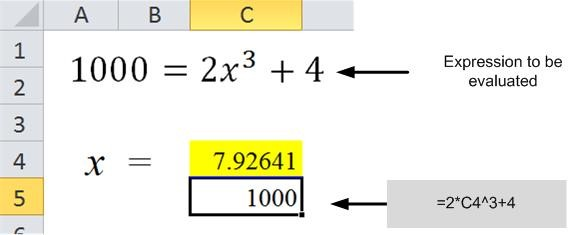Find the value of x that satisfies with Goal Seek:

The following picture shows how to setup a sheet that evaluates to give the value of x such that expression equals 1000.

The expression to be evaluated in converted into an Excel formula: 2*C4^3+4. Here C4 is the cell where iterated value will be stored. We have to put manually a the desired output (1000) in the field “To Value:” and the Set Cell should contain C5.

Once you put the ok key, the dialogue box will evaluate to the appropriate value of x, which for this case is 7.926.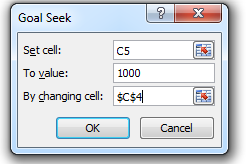Example # 2:

Find the break-even volume with Fixed Cost of \$1000 and Variable cost of \$1.5/Unit.

The picture on the right shows how the sheet is setup for this situation. The Formula for total cost is TC = FC + Volume x Unit Price. In order to reach the break even, the total cost must equal to the sales volume. The sales volume equals Total Units sold times the unit sales price.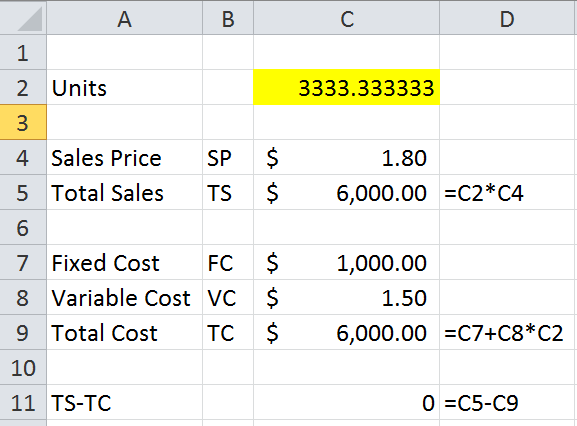When we put all this information in to the goal-seek dialogue box, we reach the figure of 3333.33 Units that can be rounded up to 3334 Units.

Thus we can find the breakeven values with Goal Seek as well.